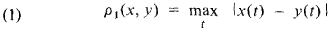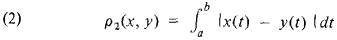# metric space

Also found in: Dictionary, Thesaurus, Wikipedia.
Related to metric space: Complete metric space

## metric space

[′me·trik ′spās]
(mathematics)
Any topological space which has a metric defined on it.
McGraw-Hill Dictionary of Scientific & Technical Terms, 6E, Copyright © 2003 by The McGraw-Hill Companies, Inc.
The following article is from The Great Soviet Encyclopedia (1979). It might be outdated or ideologically biased.

## Metric Space

a set of objects (points) in which a metric is introduced. Any metric space is a topological space; all possible open spheres are taken as neighborhoods in the space; in this case, the set of all points x for which the distance p(x, xo) < K is said to be an open sphere of radius K with center at the point XD- The topology of a given set may vary as a function of the metric introduced in it. For example, the following two metrics may be introduced in the set of real functions that are defined and continuous in the interval [a, b] of the number axis:The corresponding metric spaces have different topological properties. A metric space with metric (1) is complete [for any sequence of its points {xn} such that ρ1(xn, xm) → 0 as n, m → ∞, we can find an element x0 of the metric space that is the limit of this sequence]; a metric space with metric (2) does not have this property. Fundamental concepts of analysis can be introduced in a metric space, such as the continuity of the mapping of one metric space into another, convergence, and compactness. The concept of metric space was introduced by M. Fréchet in 1906.

### REFERENCES

Aleksandrov, P. S. Vvedenie v obshchuiu teoriiu mnozhestv i funktsii. Moscow-Leningrad, 1948.
Kolmogorov, A. N., and S. V. Fomin. Elementy teorii funktsii i funktsional’nogo analiza, 3rd ed. Moscow, 1972.
Liusternik, L. A., and V. I. Sobolev. Elementy funktsionaVnogo analiza, 2nd ed. Moscow, 1965.

V. I. SOBOLEV

The Great Soviet Encyclopedia, 3rd Edition (1970-1979). © 2010 The Gale Group, Inc. All rights reserved.

## metric space

(mathematics)
A set of points together with a function, d, called a metric function or distance function. The function assigns a positive real number to each pair of points, called the distance between them, such that:

1. For any point x, d(x,x)=0;

2. For any two distinct points x and y, d(x,y)>0;

3. For any two points x and y, not necessarily distinct,

d(x,y) = d(y,x).

4. For any three points x, y, and z, that are not necessarily distinct,

d(x,z) <= d(x,y) + d(y,z).

The distance from x to z does not exceed the sum of the distances from x to y and from y to z. The sum of the lengths of two sides of a triangle is equal to or exceeds the length of the third side.
This article is provided by FOLDOC - Free Online Dictionary of Computing (foldoc.org)
References in periodicals archive ?
A function d : X x X [right arrow] R is an asymmetric metric and (X, d) is an asymmetric metric space if
It is clear that the metric space (A, d) is complete.
In Section 2; we give definitions of NT set , NT metric space  and NT topology .
 Let (X, d) be a complete metric space and let T: X [right arrow] X be a Reich type single-valued (a, b, c)-contraction, that is, there exist non-negative numbers a, b, c with a + b + c < 1 such that
Let (X, d) be a complete metric space and [f.sub.1], ..., [f.sub.N] be weak contractions.
Let X be a complete metric space and let [PHI] : X [right arrow] [(IFS).sup.X], [psi] : X [right arrow] X be ([alpha], [beta]) compatible mappings.
A metric space (X, d) endowed with a digraph G such that V (G) = X is denoted by (X, d, G).
Let (X, d) be a generalized metric space, let {[x.sub.n]} [subset] X be a Cauchy sequence, and x, y [member of] X.
In , the notion of a rectangular metric space was extended to rectangular partial metric spaces as follows.
If we take s = 1, the definition reduces to the [lambda]-quasi-contraction in the setting of metric spaces.
Let (X, d) be a complete metric space and let T be a self-mapping on X satisfying (2).
Let (X, [rho]) be a metric space with a metric [rho].

Site: Follow: Share:
Open / Close